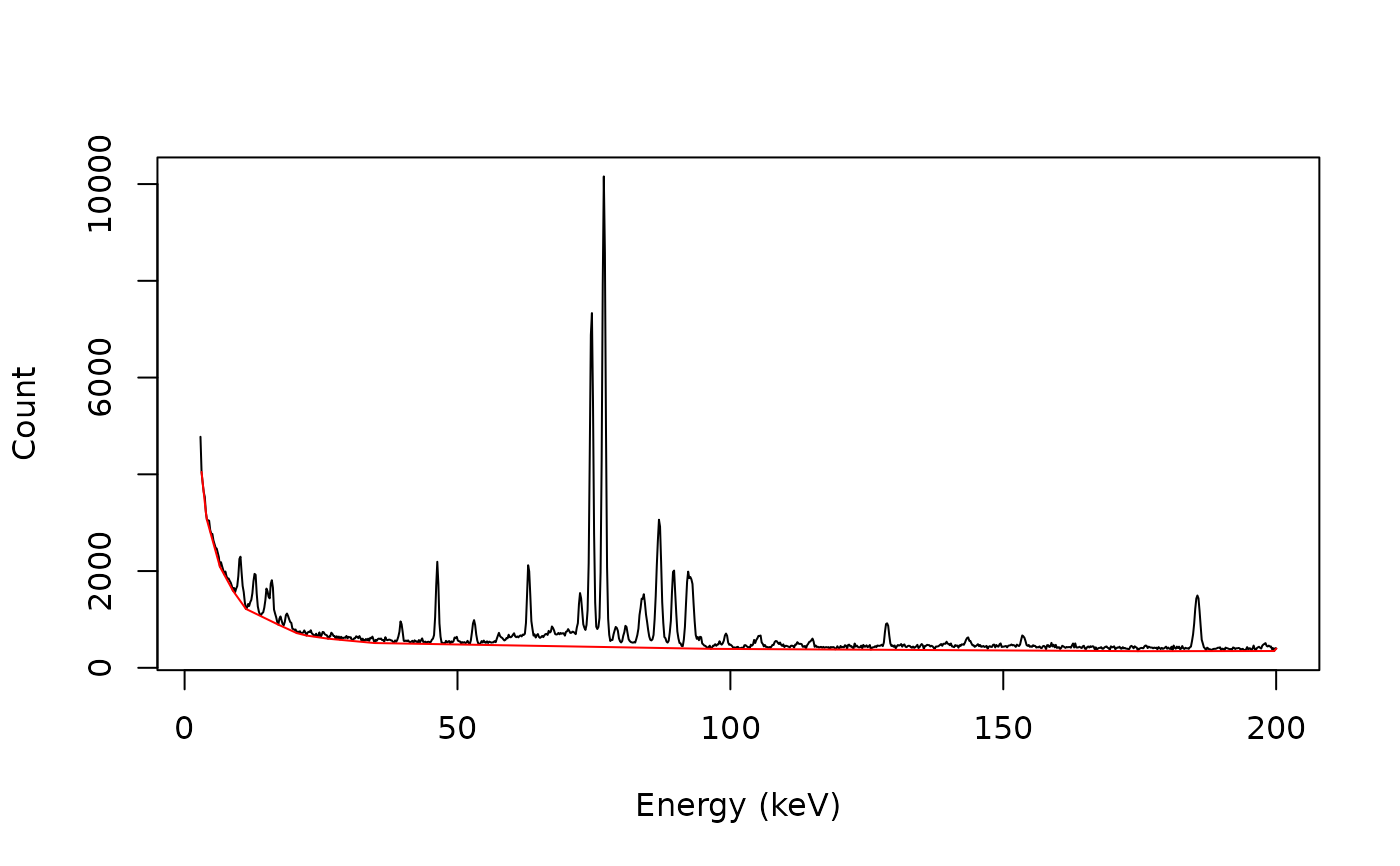Rubberband Baseline Estimation

## Usage

baseline_rubberband(x, y, ...)

# S4 method for numeric,numeric
baseline_rubberband(x, y, noise = 0, spline = TRUE, ...)

# S4 method for ANY,missing
baseline_rubberband(x, noise = 0, spline = TRUE, ...)

## Arguments

x, y

A numeric vector. If y is missing, an attempt is made to interpret x in a suitable way (see grDevices::xy.coords()).

...

Extra arguments to be passed to stats::smooth.spline().

noise

A length-one numeric vector giving the noise level. Only used if method is "rubberband".

spline

A logical scalar: should spline interpolation through the support points be used instead of linear interpolation? Only used if method is "rubberband".

## Value

Returns a list with two components x and y.

## Details

A convex envelope of the spectrum is determined and the baseline is estimated as the part of the convex envelope lying below the spectrum. Note that the rubber band does not enter the concave regions (if any) of the spectrum.

## Note

baseline_rubberband() is slightly modified from C. Beleites' hyperSpec::spc.rubberband().

signal_correct()

Other baseline estimation methods: baseline_linear(), baseline_peakfilling(), baseline_polynomial(), baseline_rollingball(), baseline_snip()

N. Frerebeau

## Examples

## gamma-ray spectrometry
data("BEGe")

## Subset from 2.75 to 200 keV
BEGe <- signal_select(BEGe, from = 3, to = 200)

## Plot spectrum
plot(BEGe, type = "l", xlab = "Energy (keV)", ylab = "Count")

## Rubberband baseline
baseline <- baseline_rubberband(BEGe)

plot(BEGe, type = "l", xlab = "Energy (keV)", ylab = "Count")
lines(baseline, type = "l", col = "red")